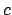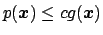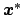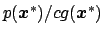Next: Importance sampling Up: Monte Carlo methods Previous: Estimating expectations by sampling

### Rejection sampling

Let us assume we are able to generate points according to some function, such that, given a constant,. We generateaccording toand decide to accept it with probability(i.e. we extract another random number between 0 and 1 and accept the point if this number is below that ratio). It is easy to show that this procedure reshapestoand that it does not depend on the absolute normalization of(any normalization constant can be absorbed in the multiplicative constant). A trivial choice of, especially for simple one-dimensional problems, is a uniform distribution (this variation is known as the hit or miss method), though clearly it can be very inefficient.

Giulio D'Agostini 2003-05-13